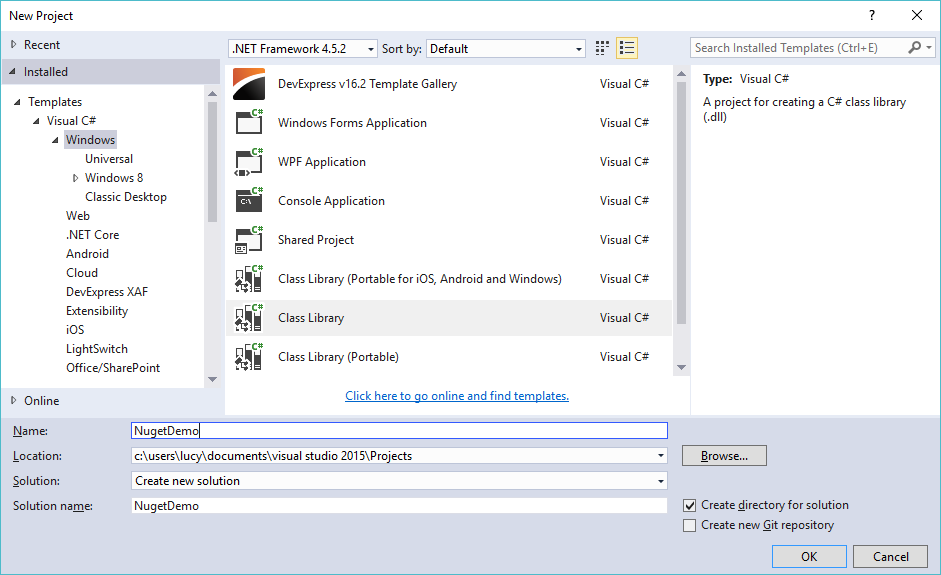# How to create a nuget package

## How to Create a nuget package in Visual Studio. Nuget is how you package that functionality into a component that can be shared and used by any developer

Step 1Click New Project, then select Visual C# on the left, then Windows and then select Class Library. Name your project "NugetDemo" and then click OKStep 2: Create a simple math class

```public class MyMath
{
public int Add(int a, int b)
{
return a + b;
}

public int Sub(int a, int b)
{
return a - b;
}

public decimal Div(int a, int b)
{
return (decimal)a / (decimal)b;
}
}```

Step 3: Create a nuget pack as below video, then add code to play demo

```class Program
{
static void Main(string[] args)
{
int a = 1;
int b = 2;
MyMath math = new MyMath();
Console.Write("a + b={0}", math.Add(a, b));
Console.WriteLine();
Console.Write("a/b={0}", math.Div(a, b));
Console.ReadKey();
}
}```

VIDEO TUTORIALS